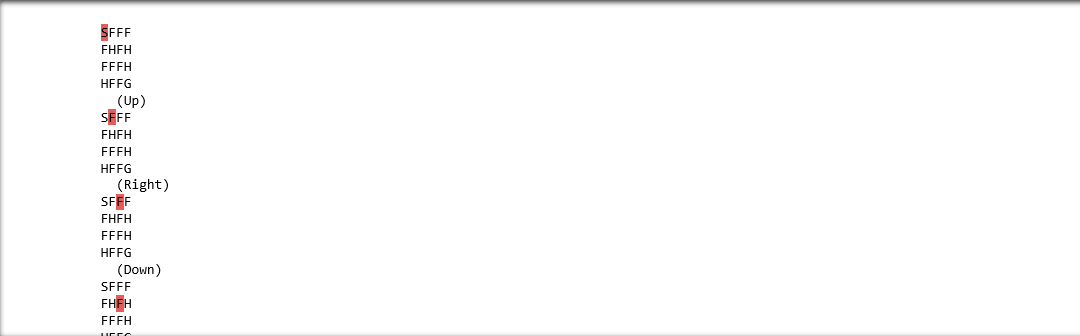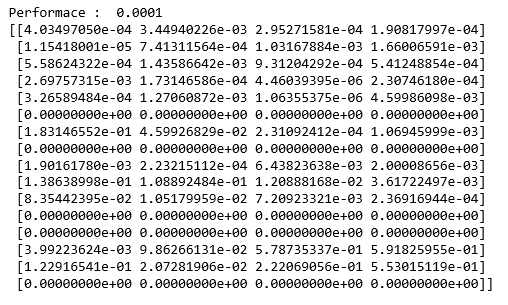Skip to content
Related Articles
SARSA Reinforcement Learning
• Last Updated : 18 Jun, 2019

Prerequisites: Q-Learning technique

SARSA algorithm is a slight variation of the popular Q-Learning algorithm. For a learning agent in any Reinforcement Learning algorithm it’s policy can be of two types:-

1. On Policy: In this, the learning agent learns the value function according to the current action derived from the policy currently being used.
2. Off Policy: In this, the learning agent learns the value function according to the action derived from another policy.

Q-Learning technique is an Off Policy technique and uses the greedy approach to learn the Q-value. SARSA technique, on the other hand, is an On Policy and uses the action performed by the current policy to learn the Q-value.

This difference is visible in the difference of the update statements for each technique:-

1. Q-Learning:2. SARSA:Here, the update equation for SARSA depends on the current state, current action, reward obtained, next state and next action. This observation lead to the naming of the learning technique as SARSA stands for State Action Reward State Action which symbolizes the tuple (s, a, r, s’, a’).

The following Python code demonstrates how to implement the SARSA algorithm using the OpenAI’s gym module to load the environment.

Step 1: Importing the required libraries

 import numpy as npimport gym

Step 2: Building the environment

Here, we will be using the ‘FrozenLake-v0’ environment which is preloaded into gym. You can read about the environment description here.

 #Building the environmentenv = gym.make('FrozenLake-v0')

Step 3: Initializing different parameters

 #Defining the different parametersepsilon = 0.9total_episodes = 10000max_steps = 100alpha = 0.85gamma = 0.95  #Initializing the Q-matrixQ = np.zeros((env.observation_space.n, env.action_space.n))

Step 4: Defining utility functions to be used in the learning process

 #Function to choose the next actiondef choose_action(state):    action=0    if np.random.uniform(0, 1) < epsilon:        action = env.action_space.sample()    else:        action = np.argmax(Q[state, :])    return action  #Function to learn the Q-valuedef update(state, state2, reward, action, action2):    predict = Q[state, action]    target = reward + gamma * Q[state2, action2]    Q[state, action] = Q[state, action] + alpha * (target - predict)

Step 5: Training the learning agent

 #Initializing the rewardreward=0  # Starting the SARSA learningfor episode in range(total_episodes):    t = 0    state1 = env.reset()    action1 = choose_action(state1)      while t < max_steps:        #Visualizing the training        env.render()                  #Getting the next state        state2, reward, done, info = env.step(action1)          #Choosing the next action        action2 = choose_action(state2)                  #Learning the Q-value        update(state1, state2, reward, action1, action2)          state1 = state2        action1 = action2                  #Updating the respective vaLues        t += 1        reward += 1                  #If at the end of learning process        if done:            breakIn the above output, the red mark determines the current position of the agent in the environment while the direction given in brackets gives the direction of movement that the agent will make next. Note that the agent stays at it’s position if goes out of bounds.

Step 6: Evaluating the performance

 #Evaluating the performanceprint ("Performace : ", reward/total_episodes)  #Visualizing the Q-matrixprint(Q)Attention geek! Strengthen your foundations with the Python Programming Foundation Course and learn the basics.

To begin with, your interview preparations Enhance your Data Structures concepts with the Python DS Course. And to begin with your Machine Learning Journey, join the Machine Learning – Basic Level Course

My Personal Notes arrow_drop_up